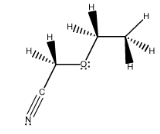# Problem: Convert the following hashed-wedged line formula into its condensed formula.

###### FREE Expert Solution

We are asked to convert the following hashed-wedged line formula into its condensed formula.

The condensed structure shows us the connectivity of the molecule.

The use of parentheses is important:

• Parentheses with no subscripts: Branch on the chain.
• Parenthesis with subscripts: Multiple branches on a chain.
• CHwithin parentheses + subscripts: Repeating CHunits within a chain.

82% (231 ratings)###### Problem Details

Convert the following hashed-wedged line formula into its condensed formula.Frequently Asked Questions

What scientific concept do you need to know in order to solve this problem?

Our tutors have indicated that to solve this problem you will need to apply the Condensed Structural Formula concept. You can view video lessons to learn Condensed Structural Formula. Or if you need more Condensed Structural Formula practice, you can also practice Condensed Structural Formula practice problems.

What professor is this problem relevant for?

Based on our data, we think this problem is relevant for Professor Smith's class at UCSD.